IEEE Vis 2018 VISxAI Workshop

# Introduction

Topology provides an alternative perspective from traditional tools for understanding shape and structure of an object. With modern advances of the computational aspects of topology, these rich theories of shape can be applied to sparse and high dimensional data, spurring the field of Topological Data Analysis (TDA). Mapper and Persistent homology are the two most popular methods in the field of TDA, but the field is nascent and rich with exciting new ideas.

The goal of this work is to introduce and explore the use of Mapper (Singh, Memoli, and Carlsson 2007). We will demonstrate a novel application of Mapper to the domain of explanatory machine learning and show how Mapper can be used to understand not just the shape of data, but also understand a machine learning model.

## Topological Building Blocks

Both Mapper and Persistent Homology make use of simplicial complexes as the tool for understanding complicated shape. A simplicial complex is a generalization of a graph, with a few special features. The most particular feature is that simplicial complexes can contain higher order analogs of vertices and edges, referred to as simplices. Simplices can be the familiar vertices and edges of a graph, or triangles drawn between 3 vertices, tetrahedron between 4 vertices, and higher still.

Most often, simplicial complexes are built from the nerve of a cover. Intuitively named, a cover of a data set is a collection of subsets of the data such that every data point is in at least one of the subsets. Formally, we say a cover {Ui} of a data set X is satisfies the condition that for any x ∈ X, there exists at least on Ui ∈ {Ui} such that x ∈ Ui. In practice, we often have that each point is contained in multiple cover elements. The nerve is a simplicial complex created from a cover by collapsing each cover element into vertices and connecting vertices when the cover elements had points in common. If a point was included in two cover elements Ui and Uj, then the vertices σi, σj would have an edge drawn between them, denoted σij. We continue this process to higher order intersections to create higher order simplices.

The image below shows a space shaped like a 2-dimensional kidney bean with a cover of 4 elements. The nerve is drawn on the right. Each cover element becomes a node of the same color, pairwise intersections become edges between those nodes, and the three-way intersections become the triangles. Because this cover was particularly nice, the resulting simplicial complex now provides a combinatorial representation of the kidney bean that is topologically equivalent to the original shape. In practice, we have little guarantee that the cover will satisfy the conditions for topological equivalence, but we can use heuristics to generate nice covers that produce close representations.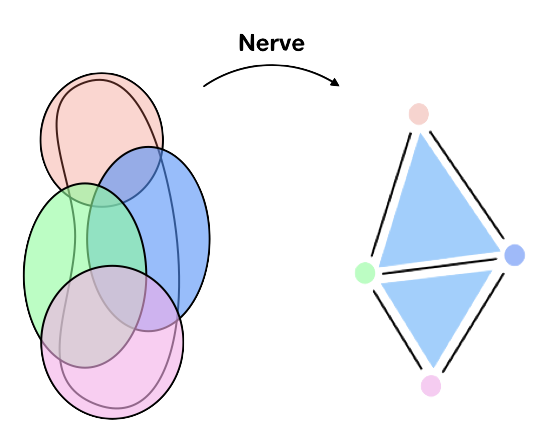The transition of topological ideas from theory to applied has been enabled by new methods for building simplicial complexes from data. Persistent Homology and Mapper are manifestations of these advances and represent two different ways to build and study covers. Persistent homology builds many different covers on the same data set and studies properties of the entire set of covers. Mapper constructs a single cover in a supervised manner and provides a representation suitable for exploratory data analysis.

In this work, we will focus on understanding the exploratory power of Mapper and how we can apply it to understand a machine learning model. For an exceptional introduction to persistent homology, we recommend (Ghrist 2008) and for other demonstrations of using Mapper for understanding a data set, see (Lum et al. 2013).

# Mapper

It is often a reasonable assumption that complex and high dimensional data exists on a much lower dimensional manifold or stratified space. Mapper can be thought of as a method of estimating and capturing this essential high dimensional structure (Singh, Memoli, and Carlsson 2007). It encodes information about relationships between regions of the data and the simplicial complex produced can be both aesthetically pleasing and useful for exploring such relationships.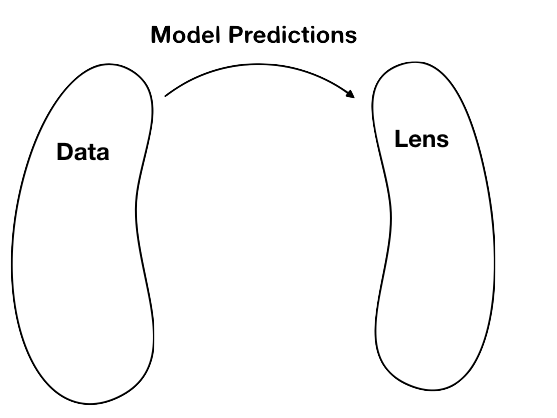The construction of Mapper makes use of two particular ideas to generate its cover, the lens and the pullback cover. The lens is a secondary representation of the data that encodes some interested aspect or information about the data set. This can be as simple as taking a subset of important columns. In this work, we interpret a machine learning model as a form of dimensionality reduction on the data, taking the predicted probabilities and decision functions as the important representation. The lens is usually where the analyst or scientist can embed domain knowledge into the construction, in our case, the representation is learned from labels. This lets the user build Mapper with respect to some information of interest and is why we say Mapper is semi-supervised.

After creating a lens, we generate a cover of this space. This cover is shown in the first image of the sequence below. In practice, the lens is chosen to be a low dimensional space, and because of this, constructing a cover is not too difficult. A common first choice of cover is to use overlapping intervals or hypercubes. We will discuss how do design a cover specifically for the output of a machine learning model a little later.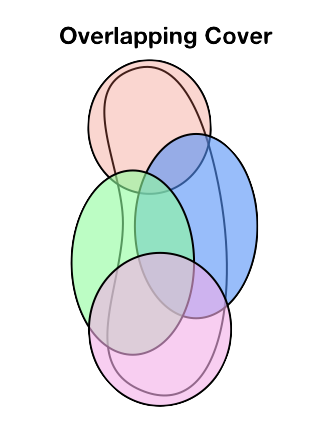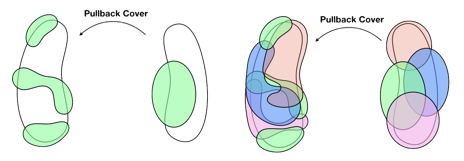A cover of the data set is found by computing the pullback cover of our constructed lens cover. The pullback of the lens cover is found by taking each cover element in the lens, finding the associated data points in the original data. Because points that are close together in the lens space are not necessarily close together in the input data space, we must refine the pulled back cover elements by applying a clustering algorithm. If our data space was a continuous space rather than discrete data, the refinement process would consist of splitting the cover element into connected components. The second image in the sequence above shows how one cover element in the lens space becomes three cover elements in the pullback cover. The third image shows the complete pullback cover of all four lens cover elements.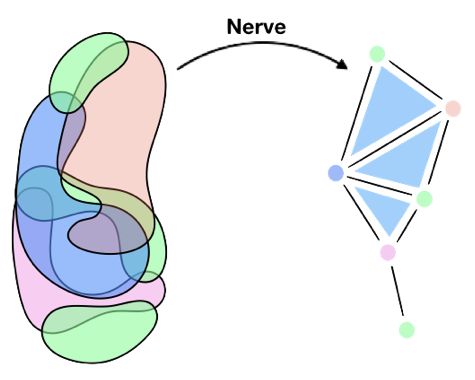The final step of Mapper is to compute the nerve of our constructed pullback cover. The result is a simplicial complex that represents the data set while capturing information about the lens representation of the data. Encoded in the simplicial complex is relationships between observations and relationships between groups of observations. These relationships are determined by nearness in the lens space and by differences in the data space. The image above shows the nerve constructed in this example.

# Data and Model

For our demonstration of applying Mapper to understand a machine learning model, we will build a classifier of leaf types using the UCI Leaf Data Set (Silva, Marcal, and Silva 2013). We chose the leaf data set because it has images included with the structured data, which is useful for illustrative purposes. As the modeling is not the emphasis of this work, we applied only a modest amount of energy into model selection and training and we are satisfied with the accuracy obtained. This is a relatively small data set and so to demonstrate that Mapper is apt for analysis of data orders of magnitude larger in both dimension and size, we will later apply Mapper to understanding a model trained on the Fashion MNIST data set (Xiao, Rasul, and Vollgraf 2017).

## Data

The leaf data set has 30 different types of leaves, but for our purposes, we will use the 10 largest classes, excluding Engl. Oak, Maple, Silver Poplar, Geranium, and Hawthorn. These five classes have very different characteristics from the rest and thus excluding them simplifies the demonstration here. The figure below shows examples from each of the 10 chosen classes. The color of the title will be used to differentiate between leaf classes in representations used later in the demonstration.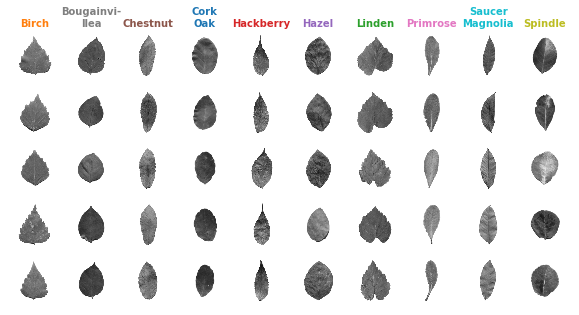The data used for our prediction model is a structured representation of these images with 14 expert engineered features such as lobedness, elongation, and smoothness. Many of the images could easily be differentiated by eye, but some classes can be easily confused.

## Model

For the classification we train a Logistic Regression model to 91.5% test set accuracy using Scikit-Learn (Pedregosa et al. 2011). Before training we applied normalization using standard scaling and applied dimensionality reduction using PCA. Using grid search and a stratified 3-fold cross validation, we found the best model using an l1 penalty with parameter c = 0.9.

# Mapper for explaining machine learning

Mapper has an often-overlooked limitation requiring the lens to have low dimension so that this space can be feasibly covered with a grid. To sample a 2-D space with a resolution of 10 cover elements per dimension requires a grid with 102 units. However, for a typical machine learning classifier with 10 classes, the same resolution grid would have 1010 units, which is computationally intractable.

To apply Mapper to understanding machine learning models, we adapted the typical cover approach by designing a new type of cover specifically for machine learning classifiers. To construct the cover, we rank and threshold the predicted probabilities for each observation and generate a cover element for similarly ranked observations. When considering a model with m classes, our approach is parameterized the top p predictions, and threshold ϵ (always taken as 1/m). We create mp cover elements, with each cover element denoted by Cij, where i is the class and j is the rank of that class in an observation’s predicted probabilities. An observation x belongs to cover element Cij if the jth highest predicted probability of x is greater than ϵ, and the class corresponding to this predicted probability is i. This allows us to work directly with the spaces generated by the model and avoid using dimensionality reduction on the prediction space.

As an example, suppose the most likely class for leaf ids #12 and #76 are both Spindle, then both leaves are added to C(Spindle), 1, Spindle’s top cover element. If the second most likely class for #12 is Chestnut, then it would be placed in C(Chestnut), 2, Chestnut’s second cover element as well. Suppose the second most likely class for #76 is Cork Oak, but the predicted probability is less than our threshold of 1/m. Then #76 will not be added to another cover element. These two observations would then produce two cover elements, one with two points and one with just one.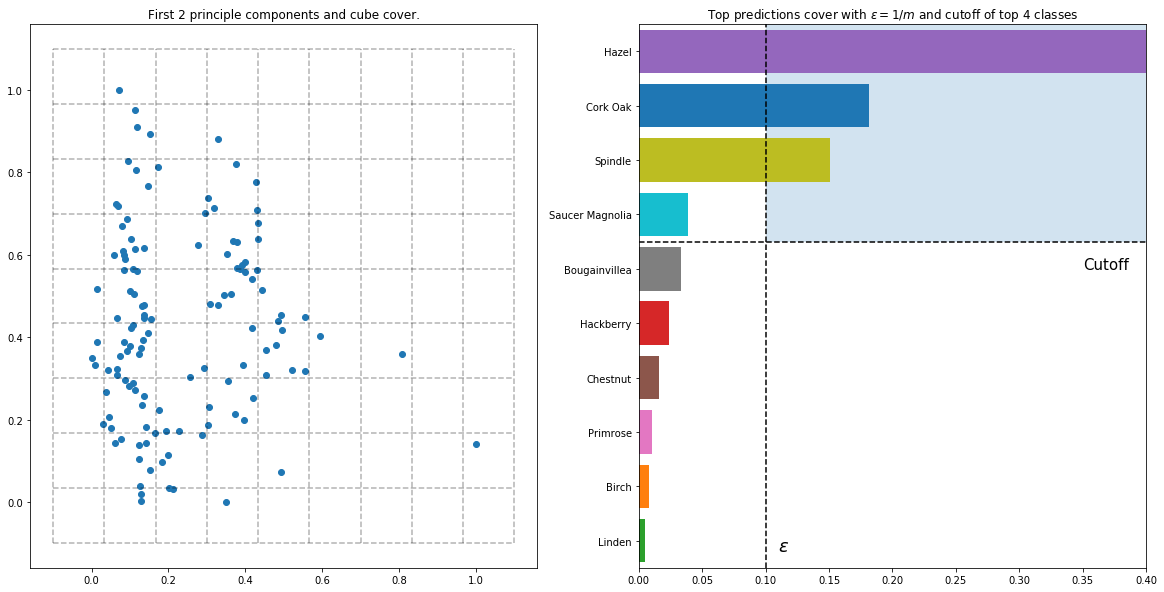The graphs above show two different ways of generating a cover of our lens. The first shows a typical approach of reducing the high dimensional space into 2 dimensions using PCA and then using a hypercube cover. To make the visualization easier to read, the cubes are shown with no overlap. Notice how many of the hypercubes in the traditional cover (on the left) are empty. In high dimensions this issue becomes even more pronounced. On the right, we demonstrate our new covering technique applied to the first observation in the data set. In this case, the leaf would be placed into 3 cover elements, C(Hazel), 1, C(Cork Oak), 2, and C(Spindle), 3.

## Instance Based Explanation

Instance based explanation is a particular type of explainable machine learning that focuses on explaining predictions through other observations. We can treat the machine learning model as a black box and explore the relationship between the input space and the prediction space using Mapper, requiring only the original data and the predicted probabilities for each observation. Because of this, the technique is generalizable to most machine learning techniques and is suitable for use by non-experts. We envision a system like what will be described would be suitable for end users to understand model predictions without needing a deep understanding of the specific modeling technique.

After looking at the global structure of Mapper, we’ll explore one particular leaf and try to use Mapper to understand what the model thinks of the leaf. We’ll introduce the idea of an escape route, a sequence of leaves that demonstrate how a leaf of interest is similar or different from the other nodes around it. After demonstrating out the routes can be used for explaining leaves, we apply the method to understanding a model trained on the Fashion MNIST data set.

## A Global View

The graph below shows the Mapper constructed using the predicted probabilities from the trained model and the particular cover described above. In this graph, the color of each node represents the majority class in that node. Because of the way the Mapper is constructed, each node represents a heterogenous set of leaves that are the model considers similar and each edge represents a relationship between nodes. Remember that because of the overlapping nature of the cover, each leaf could and probably will be in multiple different nodes.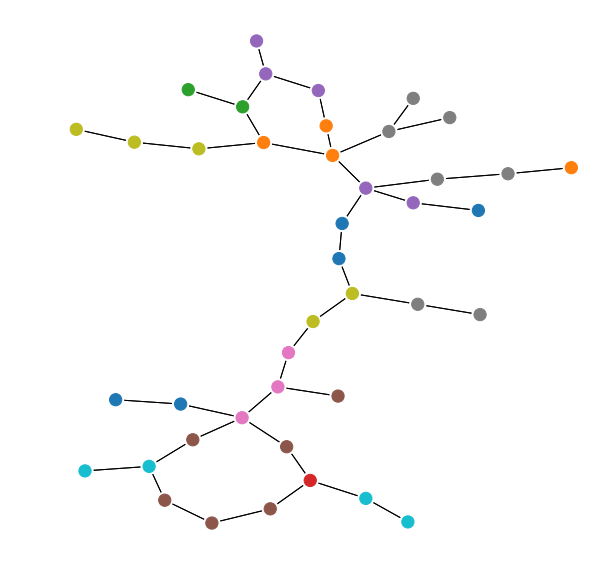In the implementation, we use Affinity Propagation clustering with damping factor of 0.5 and compute the nerve only considering 2-way intersections so edges are the highest dimensional simplices. For exploratory and exposition purposed, we’ve only kept the largest connected component of the graph. In this case, any disconnected pieces were isolated nodes.

Referring back to the grid of leaves above, we see that the pointer leaves (Hackberry, Primrose, Chestnut, and Saucer Magnolia) comprise most of the lower portion of the graph while rounder leaves (Hazel, Bougainvillea, and Birch) show up primarily at the top of the graph. The Spindle and Cork Oak join the two groups and judging from the images, do share characteristics with both.

## Local Instances

Suppose we are interested why our model decided that the particular Chestnut shown below was in fact a Chestnut. Judging from the image alone, I might have easily thought it was a Saucer Magnolia or Hazel. This particular Chestnut was chosen for demonstration because, while the classification was correct, the confidence in prediction was not very large. In fact, there were 4 classes with predicted probability greater than 1/m (with m = 10).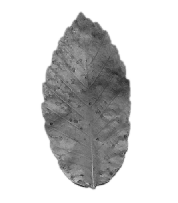We’re immediately curious which nodes of Mapper contain this Chestnut, where in the graph does this Chestnut live? In the series of graphs below, we show three different ways of visualizing the location of our Chestnut of interest. These three graphs show different levels of information about the instance. The first shows only the nodes that contain this particular Chestnut. The middle graph shows all nodes that contain any Chestnut leaves at all. The third graph shows the same region of Chestnut nodes, but also shows all of neighboring nodes, colored by their majority leaf type. The edges of the third graph are also colored based on the majority class comprising that edge.

It’s interesting that this particular leaf is in a disconnected region of Chestnuts. Maybe this one is peculiar in some way?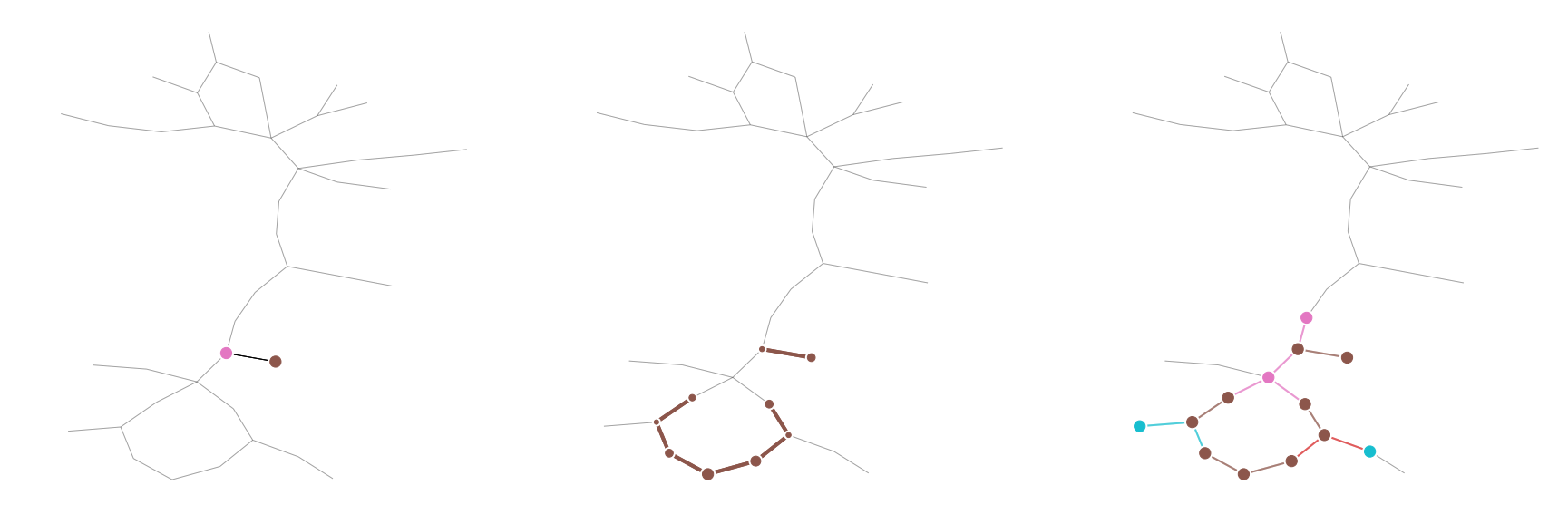Below we see all of the leaves that are in the same two nodes as the Chestnut of interest. Surprisingly, we see that the Chestnut is the only Chestnut in this subset. It is not surprising to see the Primrose and Spindle leaves in the same node, as they appear similar with an untrained eye.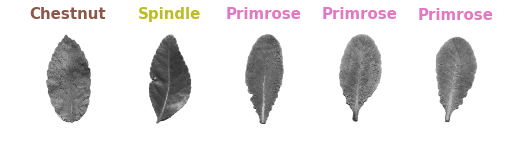## Confusion Regions

The nearby regions in the graph are one way to visualize the relationships between different observations and instances. Considering we are exploring a machine learning model, we can obtain more specific information from the Mapper as well. Remember that there were 4 different classes that had relatively high predicted probability on this Chestnut. Maybe these regions of Mapper can tell us something about the observation?

The table below shows example leaves from the 4 top predicted classes. These are Chestnut, Cork Oak, Primrose, and Spindle. While some of the Primrose have a very distinct tail or circular shape, by looking at multiple examples from the class, we can see some representatives that could cause confusion. We’ll dig much deeper into this idea while looking at escape routes.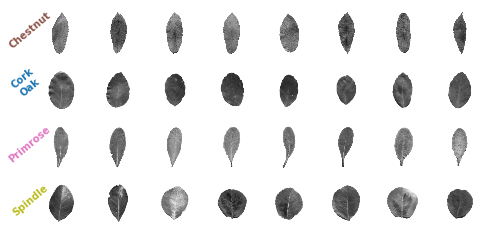The graphs below show the Mapper regions for each of the 4 classes shown above. The color of the node matches the labels in the grid above.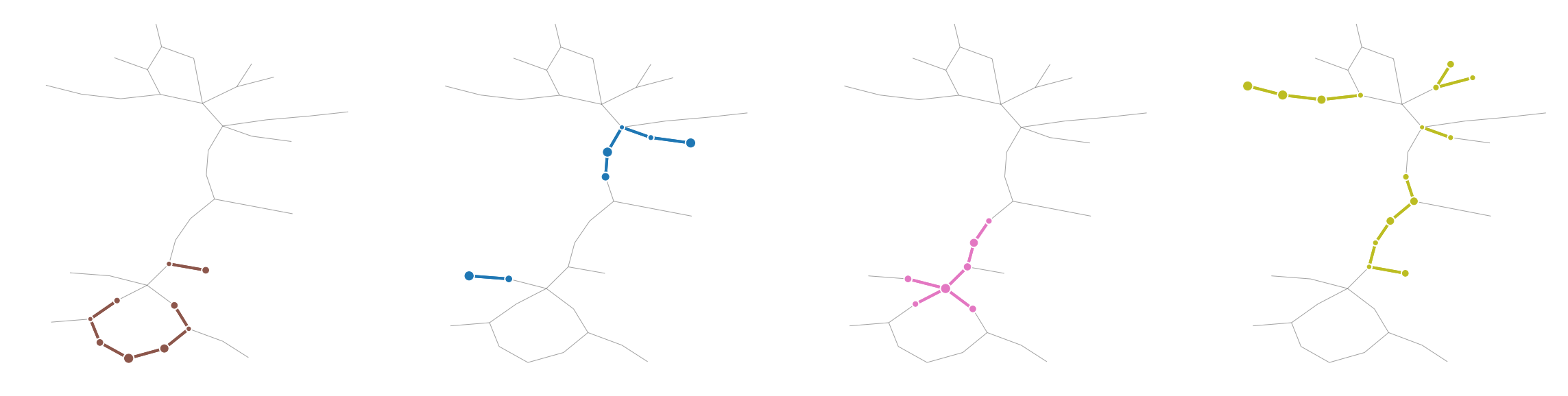## Escape Routes

Escape routes are way of exploring how a particular class or observation is related to another class by visualizing the sequence of nodes and edges between them in the Mapper. Each class creates a region in the graph, and by drawing paths between regions, we see gradual transformations. An escape route is a short path through the topological space starting in a region dominated by one class and terminating at a node that contains no observations of that class. This allows the user to understand how subtle changes to the classifier’s input effects the model’s output prediction.

An escape route is calculated by finding the shortest path tree starting at a given node in a region. The tree is pruned to exclude all hops occurring after the path has entered other regions. We define the starting region as a subgraph of the topology containing all nodes with observations whose ground truth label is the same as the Chestnut of interest. Terminating regions are regions connected to the start region containing no observations of the user selected class. Escape routes allow us to present the user with example observations, allowing the user explanation to be conveyed in terms of the underlying observations, instead of a more abstract statistical or feature-based representations.

Below, we begin exploring escape routes by first looking at the neighboring regions to the Chestnut class to gain a sense for what classes are deemed similar based on the lens and covers. These neighbors are found by first finding all nodes that have at least one Chestnut, then considering the majority class from each of the neighboring nodes containing no Chestnut instances.

The figures below show the Chestnut region, along with the 3 adjacent classes, Saucer Magnolia, Primrose, and Hackberry. Above the graphs is one representative of each class chosen at random, for illustrative purposes.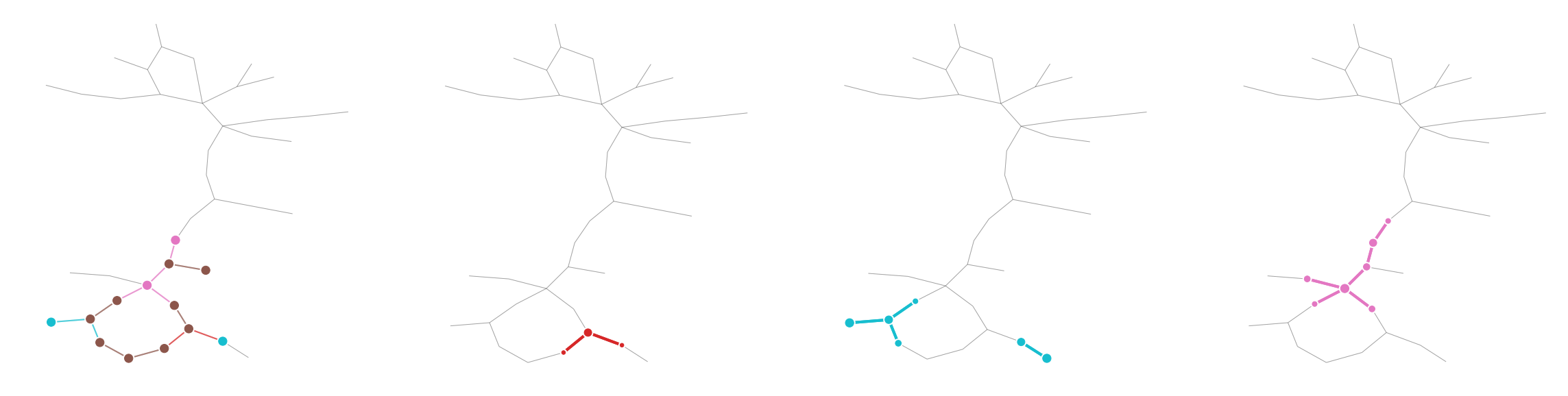The figure below shows many more examples from each of the neighbor classes. When viewing many of them, it’s easy to see their similarities. Only the most teardrop-like Hackberry and Primrose would be easy for the untrained eye to correctly classify.